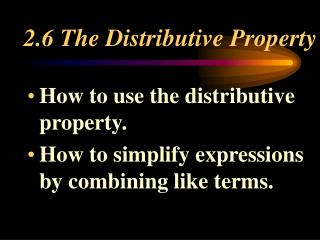DownloadDownload Presentation2.6 The Distributive Property

# 2.6 The Distributive Property

Download Presentation## 2.6 The Distributive Property

- - - - - - - - - - - - - - - - - - - - - - - - - - - E N D - - - - - - - - - - - - - - - - - - - - - - - - - - -
##### Presentation Transcript

1. 2.6 The Distributive Property • How to use the distributive property. • How to simplify expressions by combining like terms.

2. What is the Distributive property? The distributive property is one of the most important properties in Algebra. Why?

3. Why? We can use the Distributive Property to simplify expressions and make calculations easier. How?

4. How? First, we will derive the distributive property from an application. Then, we will take a look at some examples.

5. Application Kearney’s community is growing rapidly. Experts agree that space for an outdoor amusement park is limited.

6. Two architects get into an argument about the space available. One says it would be better to part the land into two parts for use. The other architect argues that keeping the land in one piece would maximize the area available.

7. Who is right? Let’s do the Math!

8. How can we figure the area of a rectangle? A = length x width Let’s examine the areas of the two proposals.

9. Area of One Rectangle Area of Two Rectangles x + 2 x 2 3 3 A1 = 3(x + 2) A2 = 3(x) + 3(2)

10. A1 = A2 Because both ways produce the same area, we can conclude that 3(x +2) = 3(x) + 3(2)

11. The Distributive Property I. The product of a and (b+c) is given by a (b + c) = ab + ac or (b +c)a = ba +ca 3(x + 2) = 3 • x + 3 • 2 (x + 2) • 3 = x • 3 + 2 • 3

12. II. The product of a and (b - c) is given by a(b - c) = ab - ac or (b - c)a = ba - ca 5(x-7) = 5• x - 5 • 7 or (x-7)5 = x • 5 - 7 • 5

13. Examples Mr. Frank will model part of today’s assignment as examples.

14. Today’s assignment • p. 98, 1 - 44 • p. 99, 51 - 54 ; Show what your answer is, why you gave that answer and how you got it.